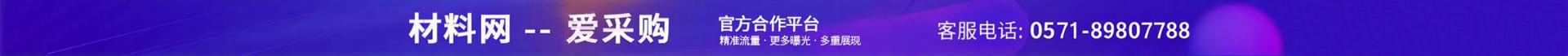•••e="3063" et="1">

pe="810585">

e="3971" et="2">

e="3984" et="3">

e="3121" et="5">